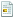2020 二月 21, 上午 04:55:59

## 游戏菜单

"限时模式"怎么样？

TC先说！

#### TombCrow

#####2006 五月 27, 上午 06:39:23 #112
030劳拉有句话：我在找一个华丽的Dias，被其他石头所包围

Grapple标准翻成什么

#### TombCrow

#####2006 五月 27, 上午 06:54:07 #115

Grapple目前是翻译成爪钩的吧？

#### 羽化蝉

#####2006 五月 27, 上午 06:54:58 #116 Last Edit: 2006 五月 31, 上午 12:04:46 by 羽化蝉

[ENSTR]="Artifacts Gallery"
[CHSTR]="古器陈列室"
// ----------------------------------
[ENSTR]="Special"
[CHSTR]="特别选项"
// ----------------------------------
[CHSTR]="手枪升级"
// ----------------------------------
[ENSTR]="Binoculars"
[CHSTR]="望远镜"
// ----------------------------------
[ENSTR]="Barrel"
[CHSTR]="桶"
// ----------------------------------
[ENSTR]="Crate"
[CHSTR]="柳条箱"
// ----------------------------------
[ENSTR]="Grapple"
[CHSTR]="抓钩"
// ----------------------------------
[CHSTR]="枪榴弹发射器"
// ----------------------------------
[CHSTR]="面具 - 铜"
// ----------------------------------
[CHSTR]="面具 - 金"
// ----------------------------------
[CHSTR]="面具 - 银"
// ----------------------------------
[ENSTR]="Pendant"
[CHSTR]="坠子"
// ----------------------------------
[ENSTR]="Pitbull"
[CHSTR]="斗牛犬"
// ----------------------------------
[ENSTR]="Puma"
[CHSTR]="美洲狮"
// ----------------------------------
[ENSTR]="Raft"
[CHSTR]="筏"
// ----------------------------------
[ENSTR]="Rifle"
[CHSTR]="步枪"
// ----------------------------------
[ENSTR]="J. Rutland"
[CHSTR]="詹姆斯·拉特兰"
// ----------------------------------
[ENSTR]="Skull Bridge"
[CHSTR]="骷髅桥"
// ----------------------------------
[ENSTR]="SMG"
[CHSTR]="冲锋枪"
// ----------------------------------
[ENSTR]="Thug B"
[CHSTR]="暴徒 乙"
// ----------------------------------
[ENSTR]="Object Models"
[CHSTR]="对象模型"
// ----------------------------------
[ENSTR]="Anaya"
[CHSTR]="安娜雅"
// ----------------------------------
[ENSTR]="Boulder"
[CHSTR]="圆石"
// ----------------------------------
[ENSTR]="Chicken"
[CHSTR]="小鸡"
// ----------------------------------
[ENSTR]="Scientist"
[CHSTR]="科学家"
// ----------------------------------
[ENSTR]="Ducati Monster"
[CHSTR]="杜卡迪怪兽摩托"
// ----------------------------------
[ENSTR]="Flare"
[CHSTR]="照明棒"
// ----------------------------------
[ENSTR]="Jeep Commander"
[CHSTR]="指挥官吉普"
// ----------------------------------
[ENSTR]="Jeep Wrangler"
[CHSTR]="牧马人吉普"
// ----------------------------------
[ENSTR]="Queen"
[CHSTR]="女王"
// ----------------------------------
[ENSTR]="Scientist"
[CHSTR]="科学家"
// ----------------------------------
[ENSTR]="Statue - Bronze"
[CHSTR]="雕像 - 铜"
// ----------------------------------
[ENSTR]="Statue - Gold"
[CHSTR]="雕像 - 金"
// ----------------------------------
[ENSTR]="Statue - Silver"
[CHSTR]="雕像 - 银"
// ----------------------------------
[ENSTR]="Shotgun"
[CHSTR]="霰弹枪"
// ----------------------------------
[ENSTR]="Transport Truck"
[CHSTR]="运输卡车"
// ----------------------------------
[ENSTR]="Turret Truck"
[CHSTR]="炮塔运载卡车"
// ----------------------------------
[ENSTR]="Truck 3"
[CHSTR]="卡车 三"
// ----------------------------------
[ENSTR]="Young Lara"
[CHSTR]="少年劳拉"
// ----------------------------------
[ENSTR]="Amanda/Entity"
[CHSTR]="阿曼达/不明怪物"
// ----------------------------------
[ENSTR]="Attack Helicopter"
[CHSTR]="武装直升机"
// ----------------------------------
[ENSTR]="Excalibur"
[CHSTR]="王者之剑"
// ----------------------------------
[ENSTR]="Excalibur Shard A"
[CHSTR]="王者之剑 碎片 甲"
// ----------------------------------
[ENSTR]="Excalibur Shard B"
[CHSTR]="王者之剑 碎片 乙"
// ----------------------------------
[ENSTR]="Excalibur Handle"
[CHSTR]="王者之剑 剑锷"
// ----------------------------------
[ENSTR]="Excalibur Hilt"
[CHSTR]="王者之剑 剑柄"
// ----------------------------------
[ENSTR]="Journal - Dais"
[CHSTR]="日记 - 祭台"
// ----------------------------------
[ENSTR]="Journal - Yetis"
[CHSTR]="日记 - 雪人"
// ----------------------------------
[ENSTR]="Obelisk"
[CHSTR]="方尖石剑台"
// ----------------------------------
[ENSTR]="Takamoto's Staff"
[CHSTR]="高本的神杖"
// ----------------------------------
[ENSTR]="Arthur"
[CHSTR]="亚瑟"
// ----------------------------------
[ENSTR]="Biker Lara"
[CHSTR]="摩托装劳拉"
// ----------------------------------
[ENSTR]="Coffin"
[CHSTR]="棺材"
// ----------------------------------
[ENSTR]="Cross - Bronze"
[CHSTR]="十字架 - 铜"
// ----------------------------------
[ENSTR]="Cross - Gold"
[CHSTR]="十字架 - 金"
// ----------------------------------
[ENSTR]="Cross - Silver"
[CHSTR]="十字架 - 银"
// ----------------------------------
[ENSTR]="Diorama - Arthur"
[CHSTR]="布景 - 亚瑟"
// ----------------------------------
[ENSTR]="Diorama - Guenevere"
[CHSTR]="布景 - 圭尼维尔"
// ----------------------------------
[ENSTR]="Diorama - Lancelot"
[CHSTR]="布景 - 兰斯洛特"
// ----------------------------------
[ENSTR]="Diorama - Merlin"
[CHSTR]="布景 - 墨林"
// ----------------------------------
[ENSTR]="Diorama - Mordred"
[CHSTR]="布景 - 莫德雷德"
// ----------------------------------
[ENSTR]="Forklift"
[CHSTR]="铲车"
// ----------------------------------
[ENSTR]="Merc C"
[CHSTR]="雇佣兵 丙"
// ----------------------------------
[ENSTR]="Sea Serpent"
[CHSTR]="海蛇"
// ----------------------------------
[ENSTR]="Sword in Stone"
[CHSTR]="石中剑"
// ----------------------------------
[ENSTR]="Tech Team Van"
[CHSTR]="技术器材车"
// ----------------------------------
[ENSTR]="Ghalali Key"
[CHSTR]="加拉利之匙"
// ----------------------------------
[ENSTR]="Merc D"
[CHSTR]="雇佣兵 丁"
// ----------------------------------
[ENSTR]="Prayer Wheel - Bronze"
[CHSTR]="转经筒 - 铜"
// ----------------------------------
[ENSTR]="Prayer Wheel - Gold"
[CHSTR]="转经筒 - 金"
// ----------------------------------
[ENSTR]="Prayer Wheel - Silver"
[CHSTR]="转经筒 - 银"
// ----------------------------------
[ENSTR]="Snow Leopard"
[CHSTR]="雪豹"
// ----------------------------------
[ENSTR]="Snow Merc 1"
[CHSTR]="雪地雇佣兵 一"
// ----------------------------------
[ENSTR]="Thug A"
[CHSTR]="暴徒 甲"
// ----------------------------------
[ENSTR]="Thug B"
[CHSTR]="暴徒 乙"
// ----------------------------------
[ENSTR]="Merc B"
[CHSTR]="雇佣兵 乙"
// ----------------------------------
[ENSTR]="Thug D"
[CHSTR]="暴徒 丁"
// ----------------------------------
[ENSTR]="Winter Lara"
[CHSTR]="冬装劳拉"
// ----------------------------------
[ENSTR]="Alister"
[CHSTR]="阿利斯特"
// ----------------------------------
[ENSTR]="Athena Statue"
[CHSTR]="雅典娜雕像"
// ----------------------------------
[ENSTR]="Crest - Bronze"
[CHSTR]="纹章 - 铜"
// ----------------------------------
[ENSTR]="Crest - Gold"
[CHSTR]="纹章 - 金"
// ----------------------------------
[ENSTR]="Crest - Silver"
[CHSTR]="纹章 - 银"
// ----------------------------------
[ENSTR]="Fish Statue"
[CHSTR]="鱼雕像"
// ----------------------------------
[CHSTR]="克劳馥夫人"
// ----------------------------------
[ENSTR]="Merc 5"
[CHSTR]="雇佣兵 五"
// ----------------------------------
[ENSTR]="Pool Platform"
[CHSTR]="泳池吊台"
// ----------------------------------
[ENSTR]="Punching Dummy"
[CHSTR]="人形训练靶"
// ----------------------------------
[ENSTR]="Thug 5"
[CHSTR]="暴徒 五"
// ----------------------------------
[ENSTR]="Winston"
[CHSTR]="温斯顿"
// ----------------------------------
[ENSTR]="Zip"
[CHSTR]="泽普"
// ----------------------------------
[ENSTR]="???"
[CHSTR]="???"
// ----------------------------------
[ENSTR]="??? Object Models"
[CHSTR]="??? 对象模型"
// ----------------------------------
[ENSTR]="Amanda"
[CHSTR]="阿曼达"
// ----------------------------------
[ENSTR]="Energy Ball - Bronze "
[CHSTR]="能量球 - 铜"
// ----------------------------------
[ENSTR]="Energy Ball - Gold"
[CHSTR]="能量球 - 金"
// ----------------------------------
[ENSTR]="Energy Ball - Silver"
[CHSTR]="能量球 - 银"
// ----------------------------------
[ENSTR]="Unknown Entity"
[CHSTR]="未知怪物"
// ----------------------------------
[ENSTR]="Hostage A"
[CHSTR]="人质 甲"
// ----------------------------------
[ENSTR]="Hostage B"
[CHSTR]="人质 乙"
// ----------------------------------
[ENSTR]="Lancelot's Shield"
[CHSTR]="兰斯洛特盾牌"
// ----------------------------------
[ENSTR]="Mag Gun"
[CHSTR]="电磁炮"
// ----------------------------------
[ENSTR]="Merc 2"
[CHSTR]="雇佣兵 二"
// ----------------------------------
[ENSTR]="Tesla Battery"
[CHSTR]="特斯拉电池"
// ----------------------------------
[ENSTR]="Thug 3"
[CHSTR]="暴徒 三"
// ----------------------------------
[ENSTR]="Train car 1"
[CHSTR]="火车车厢 一"
// ----------------------------------
[ENSTR]="Train car 2"
[CHSTR]="火车车厢 二"
// ----------------------------------
[ENSTR]="Train car 3"
[CHSTR]="火车车厢 三"
// ----------------------------------
[ENSTR]="Train Car 4"
[CHSTR]="火车车厢 四"
// ----------------------------------
[ENSTR]="Winter Lara"
[CHSTR]="冬装劳拉"
// ----------------------------------
[ENSTR]="Model Viewer"
[CHSTR]="模型浏览器"
// ----------------------------------
[ENSTR]="Knife - Bronze"
[CHSTR]="匕首 - 铜"
// ----------------------------------
[ENSTR]="Cage"
[CHSTR]="笼子"
// ----------------------------------
[ENSTR]="Knife - Gold"
[CHSTR]="匕首 - 金"
// ----------------------------------
[ENSTR]="Grapple Disc"
[CHSTR]="抓钩吸盘"
// ----------------------------------
[CHSTR]="手榴弹"
// ----------------------------------
[ENSTR]="Dual Pistols"
[CHSTR]="双枪"
// ----------------------------------
[ENSTR]="Health Pack"
[CHSTR]="医疗包"
// ----------------------------------
[ENSTR]="Jaguar"
[CHSTR]="美洲豹"
// ----------------------------------
[ENSTR]="Lara"
[CHSTR]="劳拉"
// ----------------------------------
[ENSTR]="Machine Gun"
[CHSTR]="机枪"
// ----------------------------------
[ENSTR]="Merc A"
[CHSTR]="雇佣兵 甲"
// ----------------------------------
[ENSTR]="PDA"
[CHSTR]="PDA"
// ----------------------------------
[ENSTR]="PLS"
[CHSTR]="单兵照明光源"
// ----------------------------------
[ENSTR]="Rutland's Helicopter"
[CHSTR]="拉特兰的直升机"
// ----------------------------------
[ENSTR]="Knife - Silver"
[CHSTR]="匕首 - 银"
// ----------------------------------
[ENSTR]="Thug 1"
[CHSTR]="暴徒 一"
// ----------------------------------
[ENSTR]="Bartender"
[CHSTR]="酒保"
// ----------------------------------
[ENSTR]="Buddha - Bronze"
[CHSTR]="佛像 - 铜"
// ----------------------------------
[ENSTR]="Buddha - Gold"
[CHSTR]="佛像 - 金"
// ----------------------------------
[ENSTR]="Buddha - Silver"
[CHSTR]="佛像 - 银"
// ----------------------------------
[ENSTR]="Chest"
[CHSTR]="珍宝箱"
// ----------------------------------
[ENSTR]="Dragon"
[CHSTR]="龙"
// ----------------------------------
[ENSTR]="Ducati Superbike"
[CHSTR]="杜卡迪超级摩托车"
// ----------------------------------
[ENSTR]="Evening Lara"
[CHSTR]="晚礼服劳拉"
// ----------------------------------
[ENSTR]="Evening Lara - Alt."
[CHSTR]="晚礼服劳拉 - 备选"
// ----------------------------------
[CHSTR]="玉球"
// ----------------------------------
[ENSTR]="Nishimura"
[CHSTR]="西村"
// ----------------------------------
[ENSTR]="Helicopter"
[CHSTR]="直升机"
// ----------------------------------
[ENSTR]="Party Female A"
[CHSTR]="舞会女客 甲"
// ----------------------------------
[ENSTR]="Party Female B"
[CHSTR]="舞会女客 乙"
// ----------------------------------
[ENSTR]="Party Female C"
[CHSTR]="舞会女客 丙"
// ----------------------------------
[ENSTR]="Party Male A"
[CHSTR]="舞会男客 甲"
// ----------------------------------
[ENSTR]="Party Male B"
[CHSTR]="舞会男客 乙"
// ----------------------------------
[ENSTR]="Takamoto"
[CHSTR]="高本"
// ----------------------------------
[ENSTR]="Yakuza A"
[CHSTR]="日本黑道流氓 甲"
// ----------------------------------
[ENSTR]="Yakuza B"
[CHSTR]="日本黑道流氓 乙"
// ----------------------------------

#### TombCrow

#####2006 五月 27, 上午 07:10:32 #119
[ENSTR]="Crate"
[CHSTR]="板条箱"

[ENSTR]="Raft"
[CHSTR]="筏子"

[ENSTR]="Rifle"
[CHSTR]="步枪"

[ENSTR]="Young Lara"
[CHSTR]="青年劳拉"

[ENSTR]="Jaguar"
[CHSTR]="美洲虎"

[ENSTR]="Ducati Superbike"
[CHSTR]="杜卡迪 超级摩托车"

[ENSTR]="Evening Lara - Alt."
[CHSTR]="晚礼服劳拉----备选"